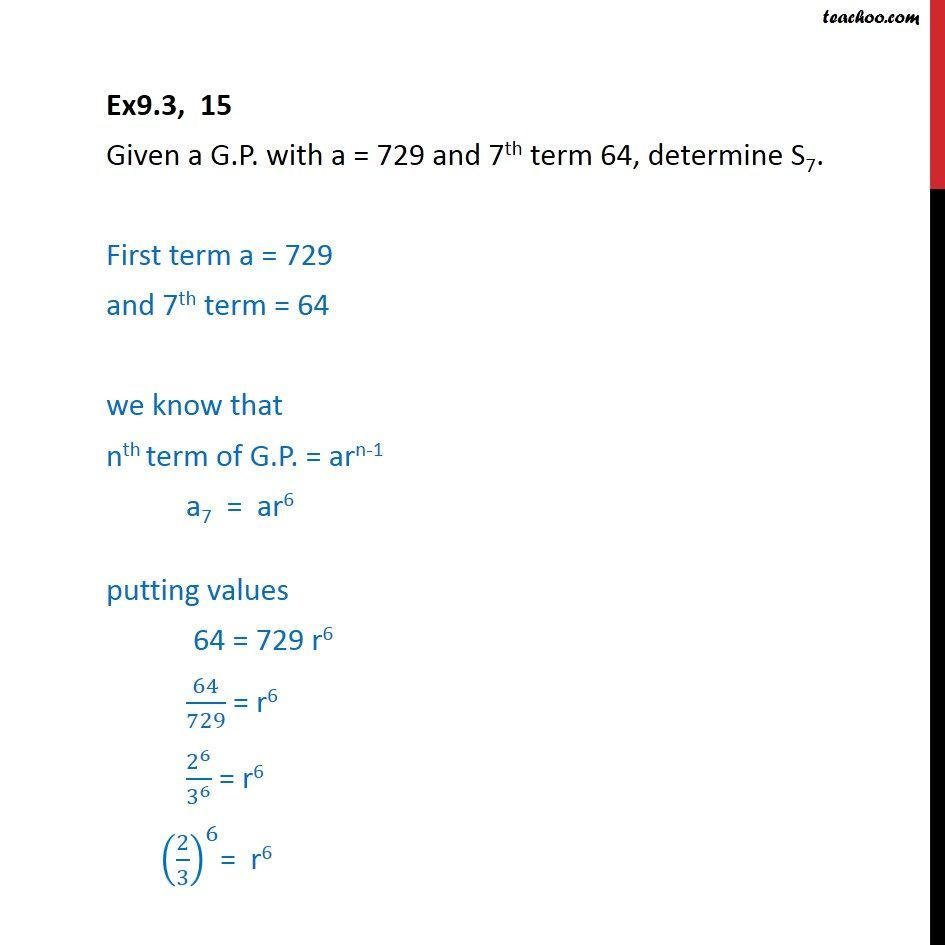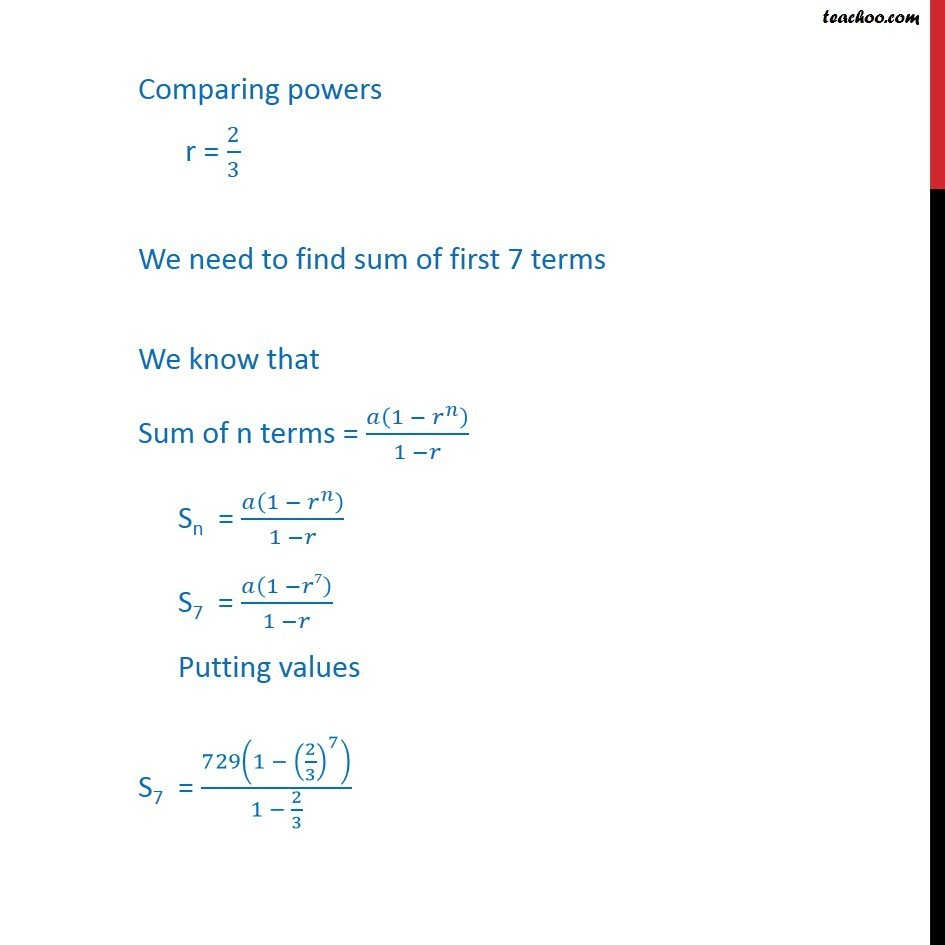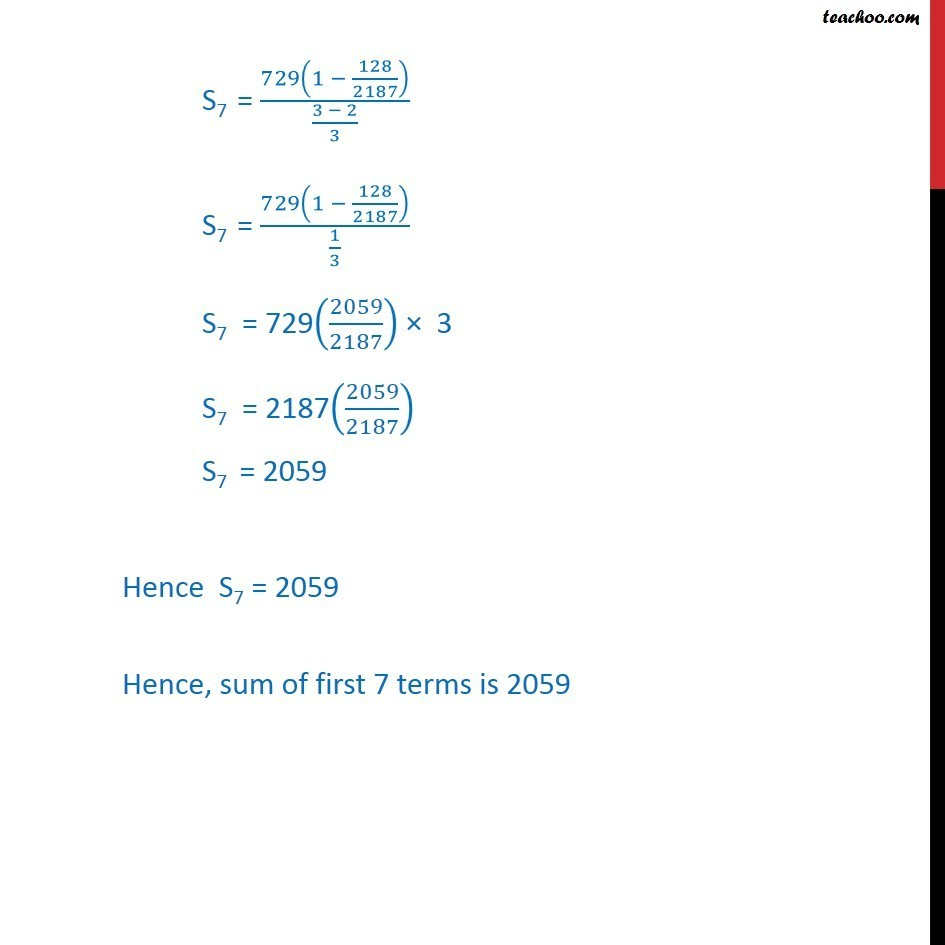Geometric Progression(GP): Formulae based

Chapter 8 Class 11 Sequences and Series
Concept wiseLearn in your speed, with individual attention - Teachoo Maths 1-on-1 Class

### Transcript

Ex9.3, 15 Given a G.P. with a = 729 and 7th term 64, determine S7. First term a = 729 and 7th term = 64 we know that nth term of G.P. = arn-1 a7 = ar6 putting values 64 = 729 r6 64/729 = r6 2^6/3^6 = r6 (2/3)^6= r6 (2/3)^6= r6 Comparing powers r = 2/3 We need to find sum of first 7 terms We know that Sum of n terms = (1 ^ )/(1 ) Sn = (1 ^ )/(1 ) S7 = (1 7)/(1 ) Putting values S7 = 729(1 (2/3)^7 )/(1 2/3) S7 = 729(1 (2/3)^7 )/(1 2/3) S7 = 729(1 128/2187)/((3 2)/3) S7 = 729(1 128/2187)/(1/3) S7 = 729(2059/2187) 3 S7 = 2187(2059/2187) S7 = 2059 Hence S7 = 2059 Hence, sum of first 7 terms is 2059